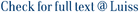We consider a classical finite horizon optimal control problem for continuous-time pure jump Markov processes described by means of a rate transition measure depending on a control parameter and controlled by a feedback law. For this class of problems the value function can often be described as the unique solution to the corresponding Hamilton-Jacobi-Bellman equation. We prove a probabilistic representation for the value function, known as nonlinear Feynman-Kac formula. It relates the value function with a backward stochastic differential equation (BSDE) driven by a random measure and with a sign constraint on its martingale part. We also prove existence and uniqueness results for this class of constrained BSDEs. The connection of the control problem with the constrained BSDE uses a control randomization method recently developed by several authors. This approach also allows to prove that the value function of the original non-dominated control problem coincides with the value function of an auxiliary dominated control problem, expressed in terms of equivalent changes of probability measures.

Constrained BSDEs representation of the value function in optimal control of pure jump Markov processes / Bandini, Elena; Fuhrman, Marco. - In: STOCHASTIC PROCESSES AND THEIR APPLICATIONS. - ISSN 0304-4149. - (In corso di stampa), pp. ---. [10.1016/j.spa.2016.08.005]

### Constrained BSDEs representation of the value function in optimal control of pure jump Markov processes

#### Abstract

We consider a classical finite horizon optimal control problem for continuous-time pure jump Markov processes described by means of a rate transition measure depending on a control parameter and controlled by a feedback law. For this class of problems the value function can often be described as the unique solution to the corresponding Hamilton-Jacobi-Bellman equation. We prove a probabilistic representation for the value function, known as nonlinear Feynman-Kac formula. It relates the value function with a backward stochastic differential equation (BSDE) driven by a random measure and with a sign constraint on its martingale part. We also prove existence and uniqueness results for this class of constrained BSDEs. The connection of the control problem with the constrained BSDE uses a control randomization method recently developed by several authors. This approach also allows to prove that the value function of the original non-dominated control problem coincides with the value function of an auxiliary dominated control problem, expressed in terms of equivalent changes of probability measures.
##### Scheda breve Scheda completa Scheda completa (DC)In corso di stampa
Backward stochastic differential equations; Marked point processes; Optimal control problems; Pure jump Markov processes; Randomization; Statistics and Probability; Modeling and Simulation; Applied Mathematics
Constrained BSDEs representation of the value function in optimal control of pure jump Markov processes / Bandini, Elena; Fuhrman, Marco. - In: STOCHASTIC PROCESSES AND THEIR APPLICATIONS. - ISSN 0304-4149. - (In corso di stampa), pp. ---. [10.1016/j.spa.2016.08.005]
File in questo prodotto:
File
FNL_Markov_REVISED_BiblioUpdated.pdf

Open Access

Tipologia: Documento in Post-print
Licenza: DRM (Digital rights management) non definiti
Dimensione 397.58 kB
Utilizza questo identificativo per citare o creare un link a questo documento: `https://hdl.handle.net/11385/172162`
•14
•14